1. /
2. CBSE
3. /
4. Class 10
5. /
6. Mathematics
7. /
8. NCERT Solutions for Class...

# NCERT Solutions for Class 10 Maths Exercise 12.1

NCERT solutions for Maths Area Related to Circles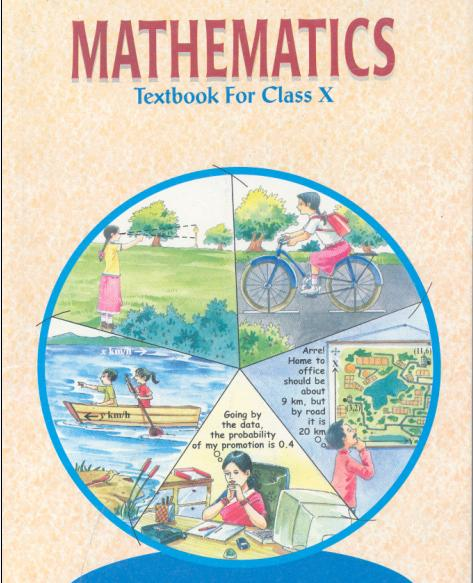## NCERT Solutions for Class 10 Maths Area Related to Circles

###### 1. The radii of two circles are 19 cm and 9 cm respectively. Find the radius of the circle which has circumference equal to the sum of the circumferences of the two circles.

Ans. Let R be the radius of the circle which has circumference equal to the sum of circumferences of the two circles, then according to question,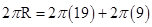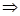R = 19 + 9R = 28 cm

NCERT Solutions for Class 10 Maths Exercise 12.1

###### 2. The radii of two circles are 8 cm and 6 cm respectively. Find the radius of the circle having area equal to the sum of the areas of the two circles.

Ans. Let R be the radius of the circle which has area equal to the sum of areas of the two circles, then

According to the question,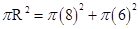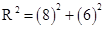R2 = 64 + 36R2 = 100R = 10 cm

NCERT Solutions for Class 10 Maths Exercise 12.1

###### 3. Figure depicts an archery target marked with its five scoring areas from the centre outwards as Gold, Red, Blue, Black and White. The diameter of the region representing Gold score is 21 cm and each of the other bands is 10.5 cm wide. Find the area of the five scoring regions.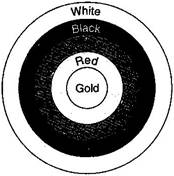Ans. Gold: Diameter = 21 cmRadius =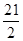cm

Area of gold scoring region =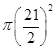=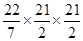= 346.5 cm2

Red: Area of red scoring region =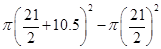=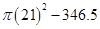=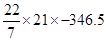= 1386 – 346.5 =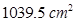Blue: Area of blue scoring region =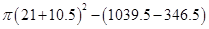=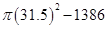=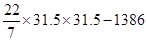= 3118.5 – 1386 =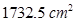Black: Area of black scoring region =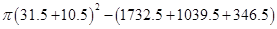=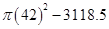=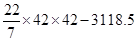= 5544 – 3118.5 =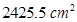White: Area of white scoring region =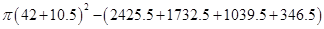=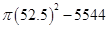=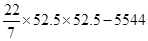= 8662.5 – 5544 =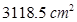NCERT Solutions for Class 10 Maths Exercise 12.1

###### 4. The wheels of a car are of diameter 80 cm each. How many complete revolutions does each wheel make in 10 minutes when the car is travelling at a speed of 66 km per hour?

Ans. Diameter of wheel = 80 cmRadius of wheel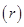= 40 cm

Distance covered by wheel in one revolution =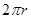=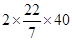=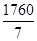cm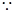Distance covered by wheel in 1 hour = 66 km = 66000 m = 6600000 cm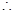Distance covered by wheel in 10 minutes =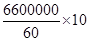= 1100000 cmNo. of revolutions =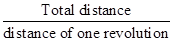=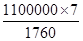= 4375

NCERT Solutions for Class 10 Maths Exercise 12.1

###### 5. Tick the correct answer in the following and justify your choice: If the perimeter and area of a circle are numerically equal, then the radius of the circle is:

(A) 2 units

(B)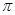units

(C) 4 units

(D) 7 units

Ans. (A) Circumference = Area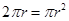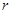= 2 units

## NCERT Solutions for Class 10 Maths Exercise 12.1

NCERT Solutions Class 10 Maths PDF (Download) Free from myCBSEguide app and myCBSEguide website. Ncert solution class 10 Maths includes text book solutions from Mathematics Book. NCERT Solutions for CBSE Class 10 Maths have total 15 chapters. 10 Maths NCERT Solutions in PDF for free Download on our website. Ncert Maths class 10 solutions PDF and Maths ncert class 10 PDF solutions with latest modifications and as per the latest CBSE syllabus are only available in myCBSEguide.

## CBSE app for Class 10

To download NCERT Solutions for Class 10 Maths, Computer Science, Home Science,Hindi ,English, Social Science do check myCBSEguide app or website. myCBSEguide provides sample papers with solution, test papers for chapter-wise practice, NCERT solutions, NCERT Exemplar solutions, quick revision notes for ready reference, CBSE guess papers and CBSE important question papers. Sample Paper all are made available through the best app for CBSETest Generator

Create question paper PDF and online tests with your own name & logo in minutes.myCBSEguide

Question Bank, Mock Tests, Exam Papers, NCERT Solutions, Sample Papers, Notes

### 8 thoughts on “NCERT Solutions for Class 10 Maths Exercise 12.1”

2. Very good and right answer